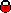## Measurement of the diffractive structure function F_2^D(3)

 Reference P Newman, talk presented at EPS 2001, July 2001 Document Conference paper Figures (1)  (10a)  (10b)  (11a)  (11b)  (12a)  (12b)  (13a)  (13b)  (14a)  (14b)  (15)  (16)  (17)  (18a)  (18b)  (18c)  (18d)  (19a)  (19b)  (19c)  (2a)  (2b)  (2c)  (2d)  (2e)  (2f)  (3)  (4)  (5)  (6)  (7a)  (7b)  (8a)  (8b)  (9a)  (9b) Tables Contact Paul Newman Abstract A new high precision inclusive measurement of the diffractive deep inelastic scattering (DIS) process ep -> eXY is presented, where Y is a proton or a low mass proton excitation carrying a fraction 1 - xpom > 0.95 of the beam longitudinal momentum and the squared 4-momentum transfer at the proton vertex t > -1 GeV^2. The measurement is presented in the form of a diffractive structure function F_2^D(3)(beta, Q^2, xpom), which is measured in the kinematic range 6.5 < Q^2 < 120 GeV^2, 0.01 < beta< 0.9 and 10^-4 <= xpom < 0.05. The xpom dependence of the data is interpreted in terms of a measurement of the effective pomeron intercept alphapom(0), which is compared with the same quantity extracted from inclusive DIS. The beta and Q^2 dependences of F_2^D are studied at fixed xpom. Scaling violations resulting in a rising dependence on ln(Q^2) are observed up to large values of beta. The Q^2 dependence of F_2^D is compared with the Q^2 dependence of F_2(x,Q^2) at the same value of x. The data can be described by the DGLAP evolution equations assuming QCD hard scattering factorisation for semi-inclusive processes and an xpom dependence motivated by Regge theory. The measured F_2^D is compared with models for diffractive DIS. Comments . Links back to overview listingH1 internal information